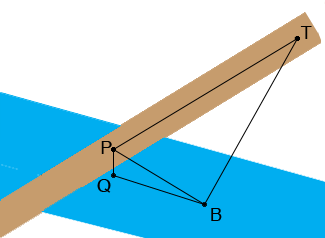SEARCH HOMEMath Central Quandaries & QueriesQuestion from Sabrina, a student: A railroad bridge is 20m above, and at right angles to, a river. A person in a train travelling at 60 km/h passes over the centre of the bridge at the same instant that a person in a motorboat travelling at 20km/h passes under the centre of the bridge. How fast are the two people separating 10s later?Hi Sabrina,

You have distance measured in metres and kilometres and time in hours and seconds. We need to decide on consistent units so I am going to measure distance in metres and time in seconds. There are 1,000 metres in a kilometre so 60 km/h is 60,000 m/h. There are 60 minutes in an hour and 60 seconds in a minute so there are 60 × 60 = 3,600 seconds on an hour. Thus 60,000 m/h is 60,000/3,600 m/s which simplifies to 100/6 m/s. Hence the train is travelling at 100/6 m/s. You can find the speed of the boat in metres per second.

In my diagram P is the position of the train and Q the position of the boat when the train is directly over the boat. T is the position of the train and B the position of the boat t seconds later.The distance from P to Q is 20 metres. Since you know the speed of the train and the boat you can find the distance from P to T and the distance from Q to B, both of these are functions of time..

Triangle PQB is a right triangle so you can use Pythagoras theorem to find the distance from P to B. Triangle TPB is also a right triangle so you can again use use Pythagoras theorem to find the distance from T to B. I am going to call this distance f(t). Your problem is to find f '(10).

I hope this helps,
HarleyMath Central is supported by the University of Regina and The Pacific Institute for the Mathematical Sciences.